# 数据结构-树

## 概念

### 定义

1. 根结点只有一个
2. 除根结点以外其他所有结点有且仅有一个前驱
3. 所有结点都可以用任意个后驱

### 术语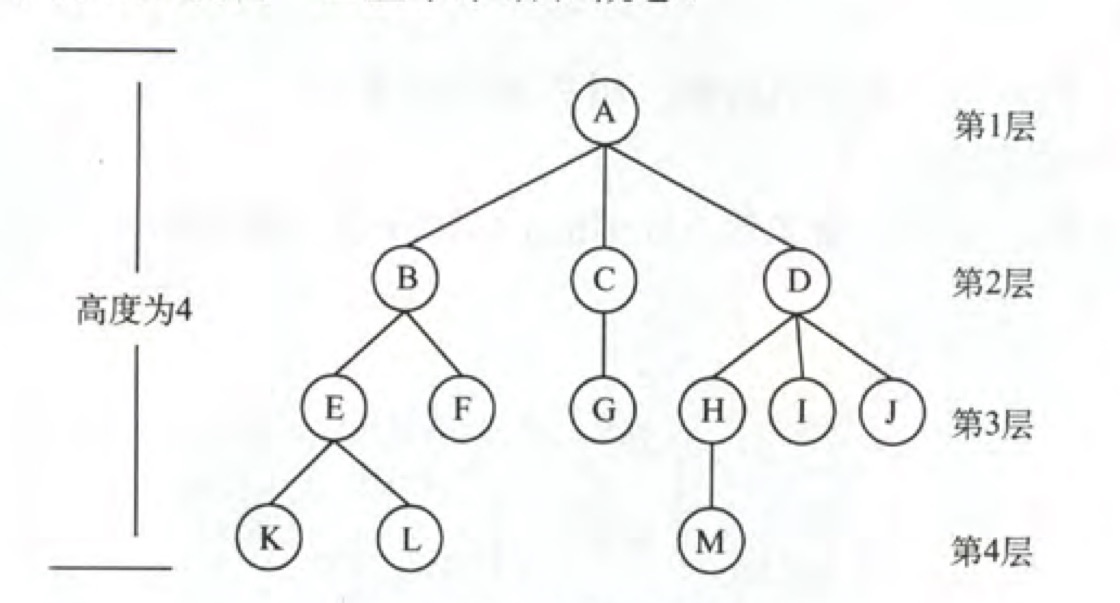• 祖先结点：结点到根结点路径上的所有前驱，A,B,E都是K的祖先结点。

• 子孙结点：结点的所有后驱，B,E,K都是A的子孙结点。

• 双亲结点：结点的直接前驱，E是K的双亲结点。

• 孩子结点：结点的直接后驱，K是E的孩子结点。

• 兄弟结点：相同双亲的结点，E,F是兄弟结点。

• 结点的度：结点的子结点个数

• 树的度：结点的最大度数

• 分支结点：度大于0的结点。

• 叶子结点：度等于0的结点。

• 结点的层次：从树根开始数层数。

• 结点的深度：自上向下。

• 结点的高度：自下向上。

• 树的高度（深度）：结点的最大层数。

• 有/无序树：结点的子树是否可以有序。

• 平衡/丰满树：除最底层，其他层都是满的。

• 森林：不相交树的集合。

### 性质

1. 树的结点数等于所有结点的度之和+1

## 二叉树

1. 最大度为2
2. 可以为空
3. 有序树

### 特殊

• 满二叉树：叶子结点都集中在最后一层的二叉树。
•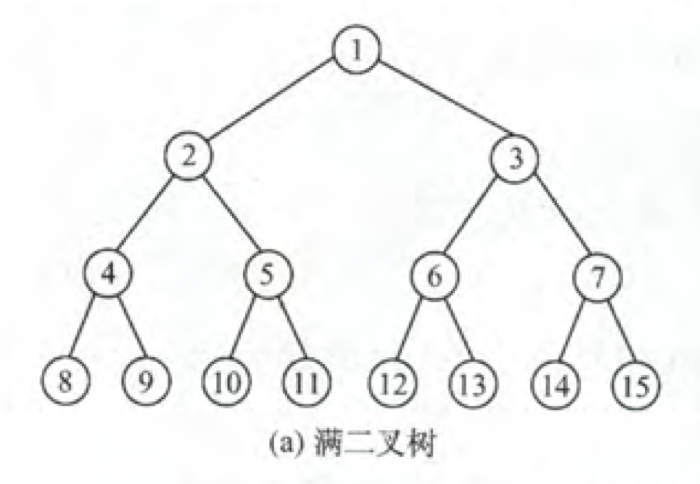• 完全二叉树：如果对满二叉树的结点进行编号，如上图所示。编号连续满二叉树子集称为完全二叉树。
•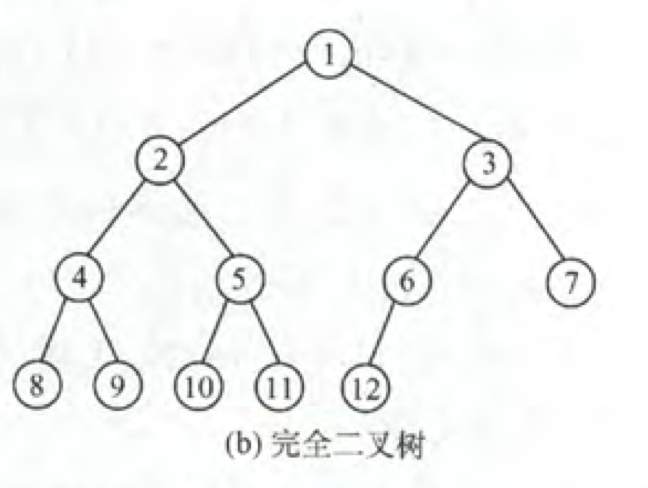• 二叉排序树：左子树结点的关键字均小于右子树的结点繁荣关键字。
• 平衡二叉树：树中任意一个结点的左右子树的深度差不超过1。

### 性质

1. 非空二叉树上的叶子结点数等于双分支结点数加1。
2. 二叉树第i层上最多有$2^{i-1}$个结点。
3. 完全二叉树对各结点从上到下，从左到右分别从1开始进行编号则对$a_i$有：
1. 若i≠1，双亲结点编号为[i/2]。
2. 若2i≤n，a左孩编号为2i，反之无左孩。
3. 若2i+1≤n，a右孩编号为2i+1，反之无右孩。
若0开始编号，双亲[i/2]-1，左孩2i+1，右孩2i+2。

### 存储

1. 顺序存储
因为我们已经知道了完全二叉树是满足一定性质的，这样即使是顺序存储也能很方便的找到其双亲和孩子结点。但是对于非完全二叉树的情况会很浪费存储空间。
2. 链式存储
typedef struct BNode{
int data;
struct BNode *lchild;
struct BNode *rchild;
}BNode, *BTree;


### 遍历

#### 递归

void PreOrder(BTree T){ //先序遍历
if(T==NULL) return;
visit(T); //访问根结点
PreOrder(T->lchild); //递归遍历左子树
PreOrder(T->rchild); //递归遍历右子树
}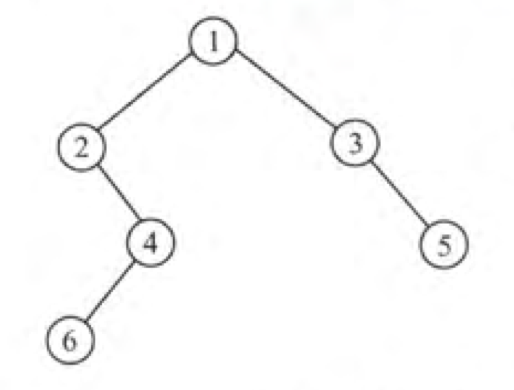• 前序：1 2 4 6 3 5
• 中序：2 6 4 1 3 5
• 后序：6 4 2 5 3 1

#### 非递归

void PreOrder(BTree T){
InitStack(S); BTree p=T;
while(p||!isEmpty(S)){
while(p){
visit(p);
stack.push(p);
p=p.lchild;
}
p=stack.pop();
p=p.rchild;
}
}


void PreOrder(BTree T){
InitStack(S); BTree p=T;
while(p||!isEmpty(S)){
while(p){
Push(S,p);
p=p.lchild;
}
Pop(S,p);
visit(p); // 弹栈后才访问根结点
p=p.rchild;
}
}


void PreOrder(BTree T){
InitStack(S); BTree p=T; BTree last=NULL;
while(p||!isEmpty(S)){
while(p){
Push(S,p);
p=p.lchild;
}
GetTop(S, p);
if(p.rchild==NULL && p==last){
visit(p);
Pop(S);
last=p;
p=NULL;
}
else{
p=p.rchild;
}
}
}


#### 层次遍历

void LevelOrder(BTree T){
InitQueue(Q); BTree p;
EnQueue(Q,T);
while(!IsEmpty(Q)){
DeQueue(Q, p);
visit(p);
if(p->lchild != NULL) EnQueue(Q, P->lchild);
if(p->rchild != NULL) EnQueue(Q, P->rchild);
}
}


#### 遍历构造

BNode* create(vector<int> &inorder, vector<int> &postorder, int is, int ie, int ps, int pe){
if(ps > pe){
return nullptr;
}
BNode* node = new BNode(postorder[pe]);
int pos;
for(int i = is; i <= ie; i++){
if(inorder[i] == node->val){
pos = i;
break;
}
}
node->left = create(inorder, postorder, is, pos - 1, ps, ps + pos - is - 1);
node->right = create(inorder, postorder, pos + 1, ie, pe - ie + pos, pe - 1);
return node;
}


def buildTree(self, inorder, postorder):
if not inorder or not postorder:
return None

root = TreeNode(postorder.pop())
inorderIndex = inorder.index(root.val)

root.right = self.buildTree(inorder[inorderIndex+1:], postorder)
root.left = self.buildTree(inorder[:inorderIndex], postorder)

return root


### 线索二叉树

#### 定义

• ptr->lchild为空，则lchild指向其中序遍历的前驱结点。
• ptr->lchild为空，则rchild指向其中序遍历的后继结点。
typedef struct ThreadNode{
int data;
int ltag, rtag;


#### 构造

void CreateInThread(ThreadTree T){
pre->rchild=NULL;
pre->rtag=1;
}

if(p!NULL){

// 线索化过程，除了线索化，其他跟普通的遍历二叉树一样
if(p->lchild==NULL){
p->lchild=pre;
p->ltag=1;
}
if(pre!=NULL&&pre->rchild==NULL){
pre->rchild=p;
pre->rtag=1;
}
pre=p;
// 线索化结束

}
}


#### 遍历

//t指向头结点，头结点左链lchild指向根结点，头结点右链rchild指向中序遍历的最后一个结点。
//中序遍历二叉线索树表示二叉树t
int InOrder(BTree T)
{
BTree *p;
*p = t->lchild;                               //p指向根结点
while(p != t)                               //空树或遍历结束时p == t
{
while(p->ltag == Link)                       //当ltag = 0时循环到中序序列的第一个结点
{
p = p->lchild;
}
printf("%c ", p->data);                      //显示结点数据，可以更改为其他对结点的操作
while(p->rtag == Thread && p->rchild != t)
{
p = p->rchild;
printf("%c ", p->data);
}

p = p->rchild;                         //p进入其右子树
}

return OK;
}



## 树与森林

### 转化1. 将同一结点的孩子串起。
2. 将每个结点的分支从左到右除第一个以外全部剪掉。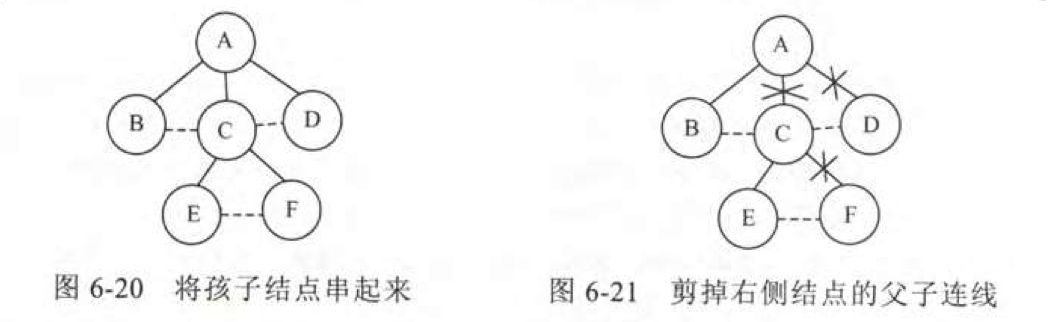1. 将二叉树从左上到右下进行斜向的分层。
2. 为每层的结点找到父结点。
3. 连接父结点，并删除层之间的结点连接。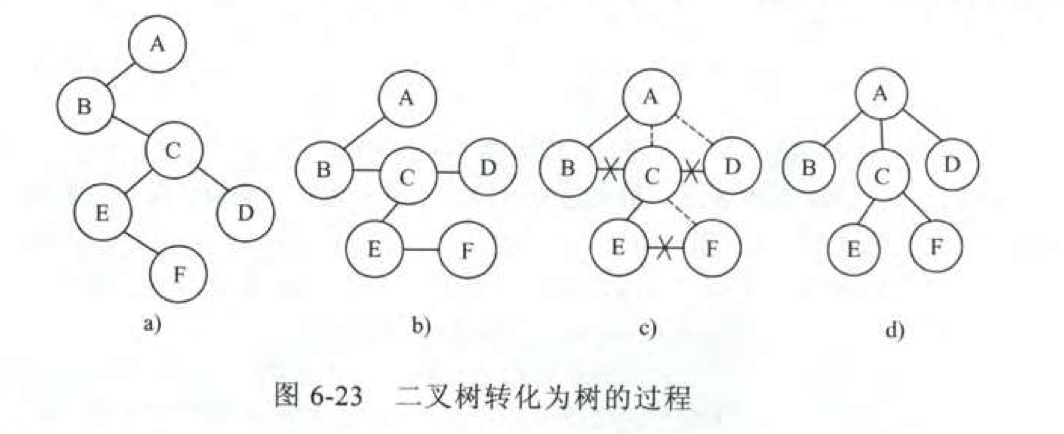1. 先将森林中的树按照树转二叉树的步骤进行二叉树转化
2. 将根结点的右孩与其他树进行拼接。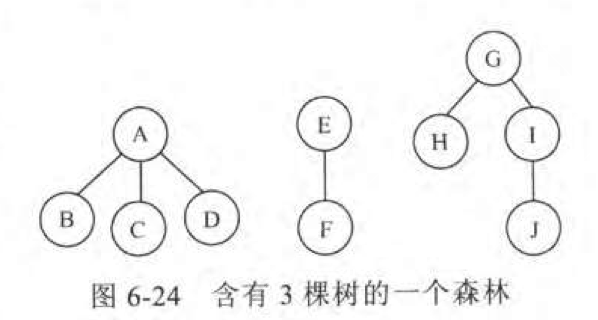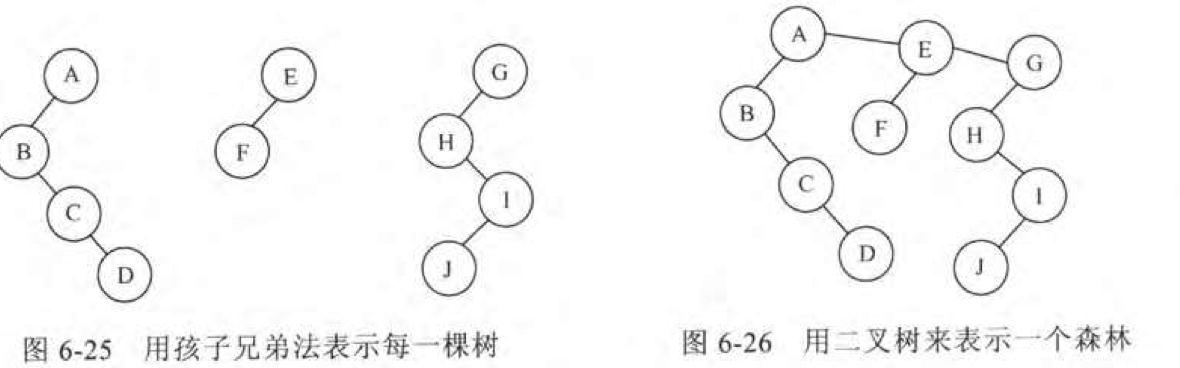1. 断开二叉树的右孩，重复此操作直到所有二叉树都没有右孩。
2. 把这些二叉树按照二叉树转树的操作转化为树

### 遍历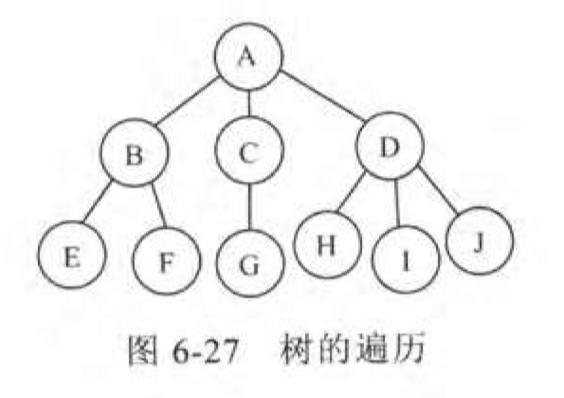## 哈夫曼树

### 概念

$WPL=\sum_{i=1}^nw_i\times l_i$

w为结点的权值，l为路径长度。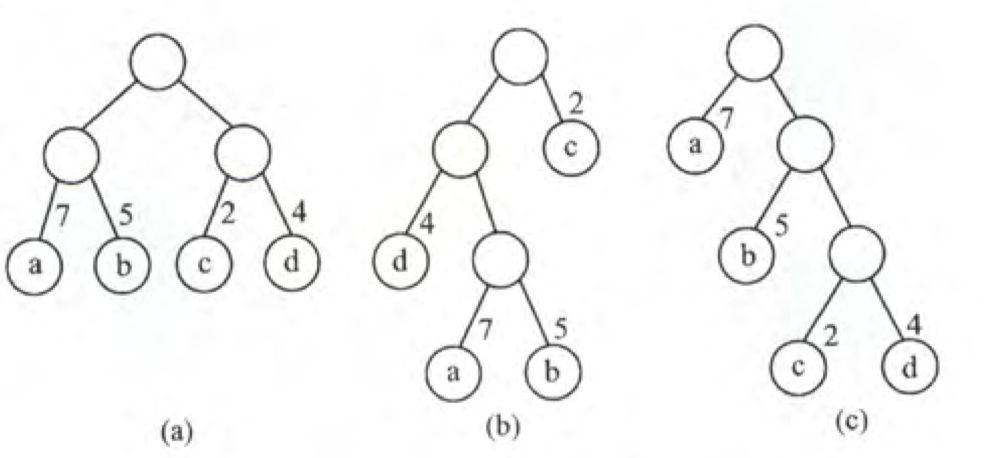a: 7x2+5x2+2x2+4x2=36
b: 7x3+5x3+2x1+4x2=46
c: 7x1+5x2+2x3+4x3=35

### 构造

1. 将这n的权值视作n棵根为n的树，记做F集合。
2. 从F选择两棵根结点权值最小的树构造新的二叉树(新的根结点的权值等于两个根结点之和)。
3. 从F删去这两个结点，并加入新结点。
4. 重复2,3直到F中只剩一棵树。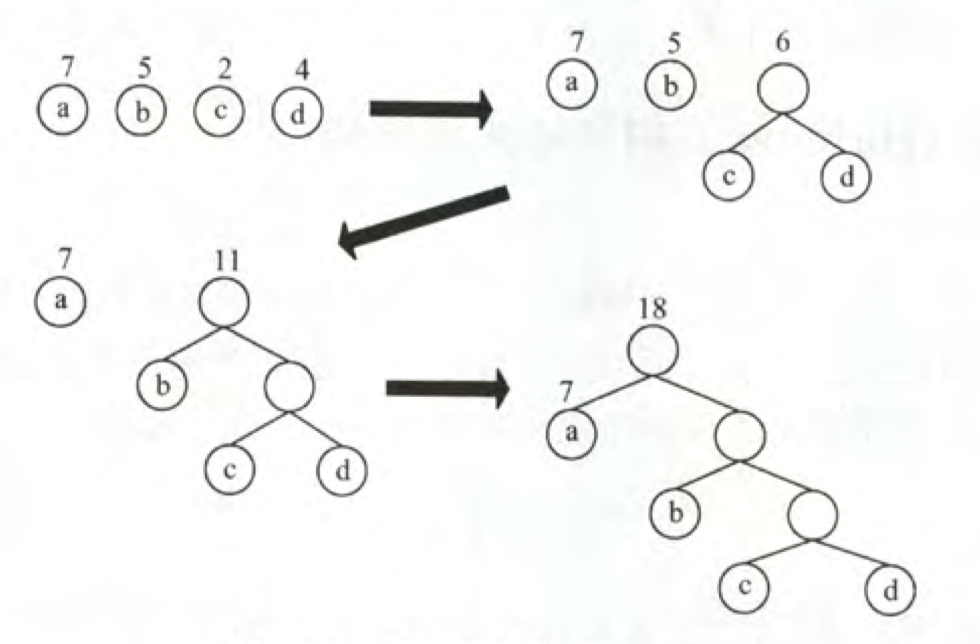1. 权值越大离根越近。
2. 没有度为1的结点，也叫正则（严格）二叉树
3. 树的带权路径最短

### 哈夫曼编码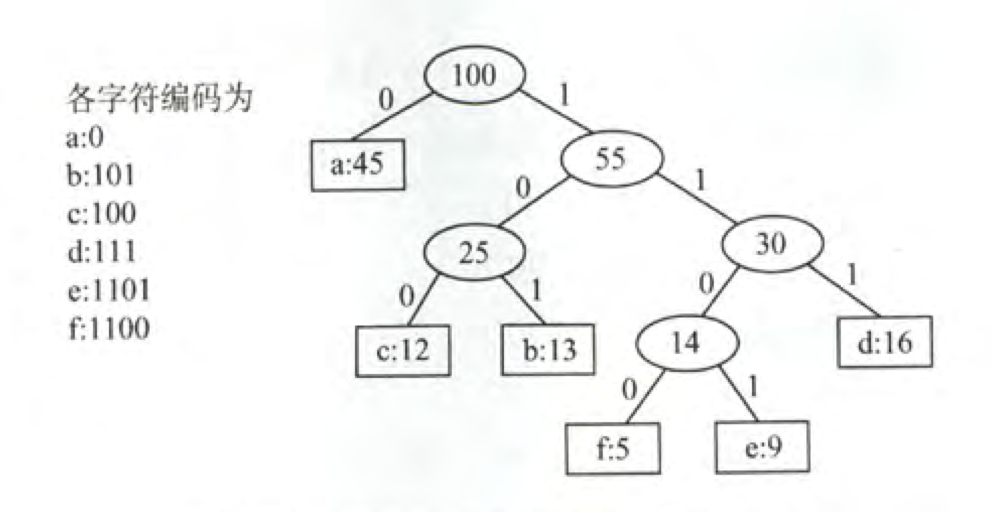## 习题

posted @ 2018-10-29 20:01  NeverMoes  阅读(2672)  评论(0编辑  收藏  举报